# Basic Concepts of Chemistry Notes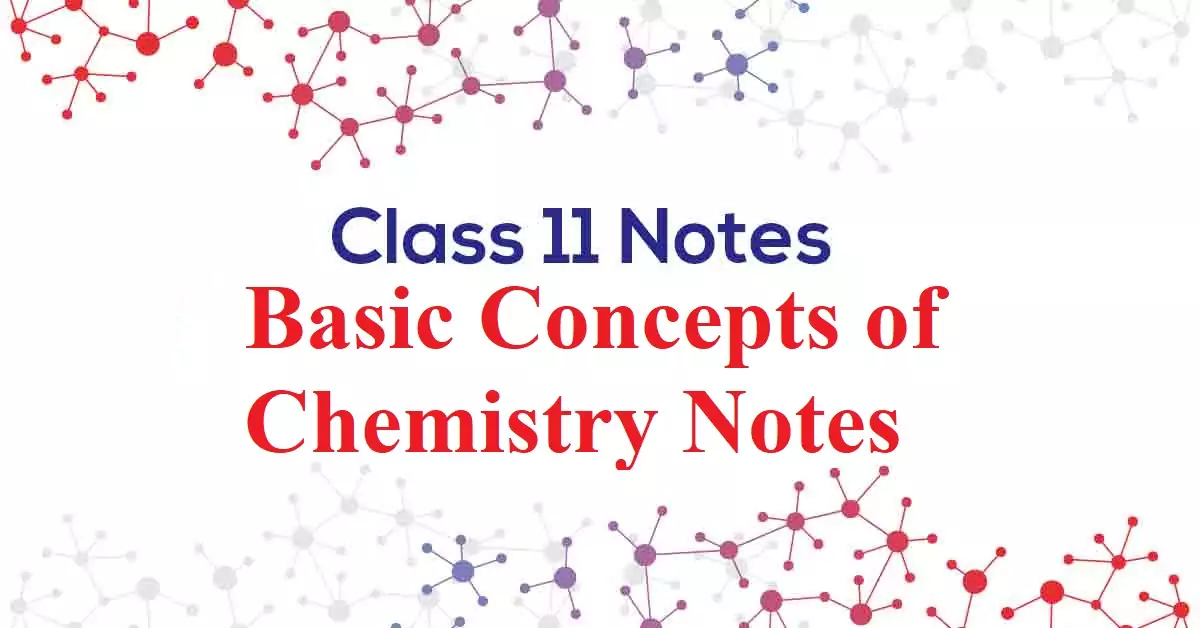## Basic Concepts of Chemistry Notes

Basic Concepts of Chemistry Notes

Atoms and Molecules: Atom is the smallest particle of an element. Molecules are the smallest particle of a substance. A molecule has all the properties of that substance.  Types of molecules Based on the type of atoms, there are two types of molecules – The homonuclear molecule and the Heteronuclear molecule. A molecule containing only one type of atom is called a Homonuclear molecule. e.g. H2, O2, N2, O3 (ozone), etc Heteronuclear molecules contain different types of atoms. E.g. CO2, H2O, C6H12O6, NH3, etc. Based on the no. of atoms there are three types of molecules – monoatomic, diatomic, and polyatomic molecules.

• Monoatomic molecules contain only one atom. E.g. all metals, noble gases like He, Ne, Ar, etc.
• Diatomic molecules contain 2 atoms. E.g. H2, O2, N2, halogens (F2, Cl2, Br2, and I2).
• Polyatomic molecules contain more than two atoms. E.g. ozone (O3), Phosphorus (P4), Sulphur (S8), etc.

Atomic Mass: It is deﬁned as the average relative mass of an atom of an element as compared to the mass of an atom of carbon 12 taken as 12.  Atomic mass is represented by u (uniﬁed mass).
Atomic mass unit (amu): 1/12th the mass of a C12 atom is called the atomic mass unit (amu).  i.e. 1 amu = 1/12 × mass of a C12 atom = 1.66 × 10-24 g = 1.66 ×10-27 kg
Today, ‘amu’ has been replaced by ‘u’ which is known as unified mass.
Gram Atomic Mass: It may be defined as the mass of 1-mole atoms of an element. For eg. Mass of one oxygen atom = 16 amu = 16/NA gms
Mass of NA Oxygen atom = 16/NA×NA = 16 gms
Average atomic mass: All most all the elements have isotopes. So, we can calculate an average atomic mass of an element by considering the atomic mass of the isotopes and their relative abundance. For e.g. Chlorine has two isotopes 35Cl and 37Cl in the ratio 3:1. So the average atomic mass Cl = (3×35 + 1×37)/4 = 35.5

Molecular mass: It is the sum of the atomic mass of the elements present in the molecule. For example: Molecular mass of CH4 = (1 × 12) + (4 × 1) = 16 u
Molecular mass of H2SO4 is calculated as: 2 × 1 + 32 + 4 × 16 = 98 u.
Mole (n): It is the amount of a substance that contains as many particles or entities as the number of atoms in exactly 12 grams of pure C-12.
1 mole of any substance contains 6.022 x 1023 atoms. This number is known as the Avogadro number or Avogadro constant (NA or N0).
1 mole of a substance = Molar mass of substance = Avogadro’s Number of chemical units = 22.4L volume at STP of a gaseous substance.
e.g., 1 mole of CH4 = 16g of CH4 = 6.022 × 1023 molecules of CH4 = 22.4L at STP.
n =   Given Mass
Molecular Mass
1 mol of hydrogen atoms = 6.022×1023 atoms
1 mol of water molecules = 6.022×1023 water molecules
1 mol of sodium chloride = 6.022 × 1023 formula units of sodium chloride

Molar volume: It is the volume of 1 mole of any substance. At standard temperature and pressure (STP), the molar volume of any gas = 22.4 L (or, 22400 mL). i.e. 22.4 L of any gas at STP contains 1 mole of the gas or 6.022 x 1023 molecules of the gas and its mass = molar mass. For e.g. 22.4 L of hydrogen gas = 1 mole of H2 = 6.022×1023 molecules of hydrogen = 2 g of H2

1 Mole of atoms = Gram atomic mass or molar mass = 6.022 x 1023 molecules = 22.4 L at NTP (00C, 1atm) = 22.7 L at STP (00C, 1 bar).

Rules in Brief: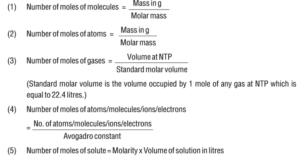Percentage composition: It is the percentage of each element present in 100g of a substance. Percentage composition (mass percent) of an element

= Mass of that element in the compound × 100
Molar mass of the compound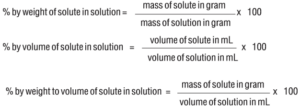Basic Concepts of Chemistry Notes

Empirical Formula: It represents the simplest whole-number ratio of various atoms present in a compound. e.g., CH is the empirical formula of benzene (C6H6).

Molecular Formula: It shows the exact number of different atoms present in a molecule of a compound. e.g., C6H6 is the molecular formula of benzene.

Relationship between empirical and molecular formulae:
Molecular formula = n × Empirical formula
Where; n = Molecular Mass
Empirical Formula Mass

Following Steps Should be Followed to Determine the Empirical Formula of the Compound:
Step 1: Conversion of mass percent of various elements into grams.
Step 2: Convert mass obtained into the number of moles.
Step 3: Divide the mole value obtained by the smallest mole value (out of the mole value of various elements calculated)
Step 4: If the ratios are not whole numbers, then they may be converted into the whole numbers by multiplying with the suitable coefficient.
Step 5: Write the empirical formula by mentioning the numbers after writing the symbols of the respective elements. For Example:

Que. A compound on analysis gave the following percentage composition Na=14.31% S=9.97% H=6.22% O=69.5%. Calculate the molecular formula of the compound on the assumption that all hydrogen in the compound is present in the combination with oxygen as the water of crystallization. The molecular mass of the compound is 322. Na=23 S=32 O=16 H=1.
Ans: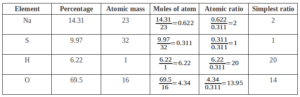Empirical Formula = Na2SH20O14
We Know
Molecular formula = n × Empirical formula
Where; n = Molecular Mass
Empirical Formula Mass
n = 322 = 1
322
Molecular Formula = 1 × Na2SH20O20 = Na2SH20O20
Final Molecular Formula – Na2SO4.10H2O

Limiting reagent (Limiting reactant): The reagent which limits a reaction or the reagent which is completely consumed in a chemical reaction is called limiting reagent or limiting reactant. For e.g. in the reaction
2SO2(g) + O2(g)    2 SO3(g), 2 moles of SO2 reacts completely with 1 mole of O2 to form 2 moles of SO3. If we take 10 moles each of SO2 and O2, we get only 10 moles of SO3 because 10 moles of SO2 require only 5 moles of O2 for the complete reaction. So here SO2 is the limiting reagent and 5 moles of O2 remain unreacted.

Basic Concepts of Chemistry Notes

Reactions in solutions:
Solutions are homogeneous mixtures containing 2 or more components. The component which is present in a larger quantities is called Solvent and the other components are called Solutes. Or, the substance which is dissolved is called the solute, and the substance in which solute is dissolved is called the solvent. For e.g. in NaCl solution, NaCl is the solute and water is the solvent. A solution containing only 2 components is called Binary Solution. If the solvent is water, it is called an aqueous solution.

Concentration Can be Expressed in the Following Ways:
Mass percent (w/w or m/m): It is defined as the number of parts solute present in 100 parts by mass of solution. i.e.
Mass % of a component =   Mass of solute  × 100
Mass of solution

Molarity (M): It is defined as the number of moles of solute dissolved per liter of solution. i.e.

Molarity (M) = Number of moles of solute (n)
Volume of the solution in litre (V)
1 M NaOH solution means 1 mole of NaOH is present in 1 L of solution.
Molarity equation:    M1V1   =    M2V2
(Before dilution) = (After Dilution)
The molarity of a solution decreases on increasing temperature. The molarity of pure water is 55.56 mol L-1.

Molality (m) = It is the number of moles of solute dissolved per 1000g (1kg) of solvent.
Molality =  Number of moles of solute
Mass of solvent in kg

Among the above concentration terms, Molarity depends on temperature because it is related to volume, which changes with temperature. Whereas Molality is Independent of Temperature.
Mole Fraction (x): It is defined as the ratio of the number of moles of a particular component to the total number of moles of solution. i.e.
Mole fraction of a component =  Number of moles of the component
Total number of moles of all the components
Let us consider we have two components 1 and 2. Therefore mole fraction of component 1 is x1, and component 2 is x2.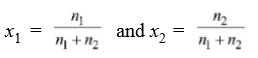x1 + x2 = 1 i.e the sum of the mole fractions of all the components in a solution is always equal to 1.

Basic Concepts of Chemistry Notes